To convert an equation of the form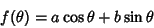(1)

to the form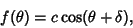(2)

expand (2) using the trigonometric addition formulas to obtain(3)

Now equate the Coefficients of (1) and (3)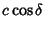(4)(5)

so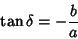(6)(7)

and we have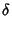(8)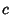(9)

Given two general sinusoidal functions with frequency: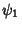(10)(11)

their sum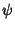can be expressed as a sinusoidal function with frequency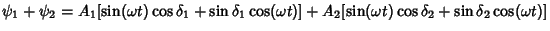(12)

Now, define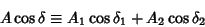(13)(14)

Then (12) becomes(15)

Square and add (13) and (14)(16)

Also, divide (14) by (13)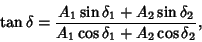(17)

so(18)

whereand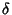are defined by (16) and (17).

This procedure can be generalized to a sum ofharmonic waves, giving(19)

where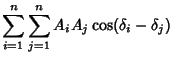(20)(21)

and(22)